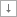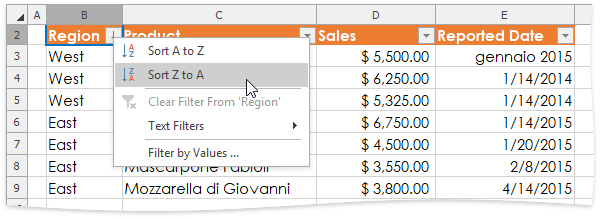# How to: Sort Data in the Filtered Range

This example demonstrates how to the sort data in the range to which the filter is applied.

1. Turn on the filtering functionality for the required range, as described in the How to: Enable Filtering example.
2. Use the AutoFilterBase.SortState property of the SheetAutoFilter object to access the SortState object, which contains basic methods to perform sorting in the filtered range. Do one of the following.

• To sort data by a single column, call the SortState.Sort method, and pass the following parameters: the zero-based index of the column to sort by, and the boolean value that specifies whether you wish to use ascending (false value) or descending (true value) sort order.

``````// Enable filtering for the specified cell range.
CellRange range = worksheet["B2:E23"];
worksheet.AutoFilter.Apply(range);

// Sort the data in descending order by the first column.
worksheet.AutoFilter.SortState.Sort(0, true);
``````

After sorting is applied, the records in the range are reordered accordingly. A tiny arrowappears in the column header to indicate that data in the filtered range are sorted by this column.• To sort data by multiple columns, create a list of the SortCondition objects, each of which defines the sort criteria to be used. To instantiate a SortCondition object, use the SortCondition constructor with the following parameters: the index of the column to sort by, and the boolean value that specifies the sort order (true, to use the descending oder; false, to sort in ascending order).

Call the SortState.Sort method and pass the list of the created SortCondition objects as a parameter. In this example, data is sorted by the first and third columns in descending order.

Note

If you sort data by multiple columns, the next sort order will be applied separately to each group of data that shares the same value in the column used in the previous sort criteria. You can sort by up to 64 columns.

``````// Enable filtering for the specified cell range.
CellRange range = worksheet["B2:E23"];
worksheet.AutoFilter.Apply(range);

// Sort the data in descending order by the first and third columns.
List<SortCondition> sortConditions = new List<SortCondition>();